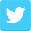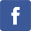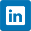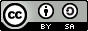A Walk Through Ethereum Classic Digital Signature Code

March 15, 2018by Christian SeberinoEthereum Classic (ETC) digital signatures secure transactions. These involve elliptic curve cryptography and the Elliptic Curve Digital Signature Algorithm (ECDSA). I will describe ETC digital signatures without these topics using only small Python functions.

BasicsSigning and verifying will be implemented using the following four constants and three functions:

N  = 115792089237316195423570985008687907852837564279074904382605163141518161494337
P  = 115792089237316195423570985008687907853269984665640564039457584007908834671663
Gx = 55066263022277343669578718895168534326250603453777594175500187360389116729240
Gy = 32670510020758816978083085130507043184471273380659243275938904335757337482424

def invert(number, modulus):
"""
Finds the inverses of natural numbers.
"""

result = 1
power  = number
for e in bin(modulus - 2)[2:][::-1]:
if int(e):
result = (result * power) % modulus
power = (power ** 2) % modulus

return result

"""
Finds the sums of two pairs of natural numbers.
"""

if   pair_1 == [0, 0]:
result = pair_2
elif pair_2 == [0, 0]:
result = pair_1
else:
if pair_1 == pair_2:
temp = 3 * pair_1 ** 2
temp = (temp * invert(2 * pair_1, P)) % P
else:
temp = pair_2 - pair_1
temp = (temp * invert(pair_2 - pair_1, P)) % P
result = (temp ** 2 - pair_1  - pair_2) % P
result = [result, (temp * (pair_1 - result) - pair_1) % P]

return result

def multiply(number, pair):
"""
Finds the products of natural numbers and pairs of natural numbers.
"""

result = [0, 0]
power  = pair[:]
for e in bin(number)[2:][::-1]:
if int(e):
result = add(result, power)
power = add(power, power)

return result

The invert function defines an operation on numbers in terms of other numbers referred to as moduli. The add function defines an operation on pairs of numbers. The multiply function defines an operation on a number and a pair of numbers. Here are examples of their usage:

>>> invert(82856, 7164661)
3032150

>>> add([84672, 5768], [15684, 471346])
[98868508778765247164450388534339365517943901419260061027507991295919394382071, 110531019976596004792591549651085191890711482591841040377832420464376026143223]

>>> multiply(82716, [31616, 837454])
[82708077205483544970470074583740846828577431856187364454411787387343982212318, 30836796656275663256542662990890163662171092281704208118107591167423888588304]

Private & Public KeysPrivate keys are any nonzero numbers less than the constant N. Public keys are the products of these private keys and the pair (Gx, Gy ). For example:

>>> private_key = 296921718

>>> multiply(private_key, (Gx, Gy))
[29493341745186804828936410559976490896704930101972775917156948978213464516647, 14120583959514503052816414068611328686827638581568335296615875235402122319824]

Notice that public keys are pairs of numbers.

SigningSigning transactions involves an operation on the Keccak 256 hashes of the transactions and private keys. The following function implements this operation:

import random

def sign(hash, priv_key):
"""
Signs the hashes of transactions.
"""

result = [0, 0]
while 0 in result or result > N / 2:
temp      = random.randint(1, N - 1)
result = multiply(temp, (Gx, Gy)) % N
result = invert(temp, N) * (hash + priv_key * result)
result = result % N

return result

For example:

>>> hash = 0xf62d00f14db9521c03a39c20e94aa10a82ff5f5a614772b25e36757a95a71048

>>> private_key = 296921718

>>> sign(hash, private_key)
[12676003675279000995677412431399004760576311052126257887715931882164427686866, 17853929027942611176839390215748157599052991088042356790746129338653342477382]

>>> sign(hash, private_key)
[18783324464633387734826042295911802941026009108876130700727156896210203356179, 41959562951157235894396660120771158332032804144867595196194581439345450008533]

Notice that digital signatures are pairs of numbers. Notice also that the sign function can give different results for the same inputs!

VerifyingVerifying digital signatures involves confirming certain properties with regards to the Keccak 256 hashes and public keys. The following function implements these checks:

def verify(sig, hash, pub_key):
"""
Verifies the signatures of the hashes of transactions.
"""

temp_1 = multiply((invert(sig, N) * hash)   % N, (Gx, Gy))
temp_2 = multiply((invert(sig, N) * sig) % N, pub_key)
sum    = add(temp_1, temp_2)
test_1 = (0 < sig < N) and (0 < sig < N)
test_2 = sum != (0, 0)
test_3 = sig == sum % N

return test_1 and test_2 and test_3

For example:

>>> hash = 0xf62d00f14db9521c03a39c20e94aa10a82ff5f5a614772b25e36757a95a71048

>>> private_key = 296921718

>>> public_key = multiply(private_key, (Gx, Gy))

>>> public_key
[29493341745186804828936410559976490896704930101972775917156948978213464516647, 14120583959514503052816414068611328686827638581568335296615875235402122319824]

>>> signature = sign(hash, private_key)

>>> signature
[54728868372105873293629977757277092827353030346967592768173610703187933361202, 18974025727476367931183775600389145833964496722266015570370178285290252701715]

>>> verify(signature, hash, public_key)
True

To verify that public keys correspond to specific ETC account addresses, confirm that the rightmost 20 bytes of the public key Keccak 256 hashes equal those addresses.

Recovery IdentifiersStrictly speaking, ETC digital signatures include additional small numbers referred to as recovery identifiers. These allow public keys to be determined solely from the signed transactions.

ConclusionI have explained ETC digital signatures using code rather than mathematics. Hopefully seeing how signing and verifying can be implemented with these tiny functions has been useful.

Feedback

You can contact me by clicking any of these icons:Acknowledgements

I would like to thank IOHK (Input Output Hong Kong) for funding this effort.